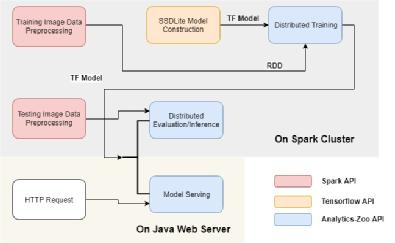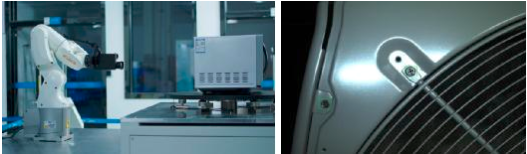## 基于Intel Analytics Zoo上分布式TensorFlow的美的/ KUKA工业检测平台

• Intel
• 2018-10-16 15:07（1）使用Spark以分布式方式处理从制造流水线获取的大量图像。

（2）使用Tensorflow Object Detection API直接构建对象检测（例如，SSDLite + MobileNet V2）模型

（3）直接使用在第一步中预处理的图像RDD，以分布式方式在Spark集群上训练（或微调）对象检测模型。

（4）直接使用评估图像集的RDD，以分布式方式在Spark集群上评估（或推断）训练模型。

（5）使用Analytics-Zoo中POJO模式的API, 将整个Pipeline 部署在低延迟的、在线Web 服务中。Spark，TensorFlow和BigDL的统一集成

<<<<

train_rdd = sc.parallelize(examples_list)

.map(lambda x: read_image_and_label(x))

.map(lambda image: decode_to_ndarrays(image))

dataset = TFDataset.from_rdd(train_rdd,

names=["images", "bbox", "classes", "num_detections"],

shapes=[[300, 300, 3],[None, 4], [None], [1)]],

types=[tf.float32, tf.float32, tf.int32, tf.int32],

batch_size=BATCH_SIZE,

hard_code_batch_size=True)

# using tensorflow object detection api to construct model

# https://github.com/tensorflow/models/tree/master/research/object_detection

from object_detection.builders import model_builder

images, bbox, classes, num_detections = dataset.tensors

detection_model = model_builder.build(model_config, is_training=True)

resized_images, true_image_shapes = detection_model.preprocess(images)

detection_model.provide_groundtruth(bbox, classes)

prediction_dict = detection_model.predict(resized_images, true_image_shapes)

losses = detection_model.loss(prediction_dict, true_image_shapes)

total_loss = tf.add_n(losses.values())

>>>>

>>>>

with tf.Session() as sess:

init_from_checkpoint(sess, CHECKPOINT_PATH)

optimizer = TFOptimizer(total_loss, RMSprop(LR), sess)

optimizer.optimize(end_trigger=MaxEpoch(20))

save_to_new_checkpoint(sess, NEW_CHEKCPOINT_PATH)

AbstractInferenceModel model = new AbstractInferenceModel(){};

model.loadTF(modelPath, 0, 0, false);

List<List<JTensor>> output = model.predict(inputs);

Midea / KUKA通过结合人工智能，计算机视觉和大数据技术，在Analytics Zoo（https://github.com/intel-analytics/analytics-zoo）上成功构建了先进的工业检测系统。它使用了工业机器人，相机和英特尔至强服务器等工具对产品的缺陷进行自动检测。尤其是Analytics Zoo提供统一的数据分析 + AI平台，可将Spark，BigDL和TensorFlow程序无缝集成到一个数据分析流水线中，从而可以轻松构建和生产化部署基于大数据的深度学习应用程序（包括分布式训练和推理，以及低延迟在线服务）。您可以参考Github上的示例了解更多详细信息。